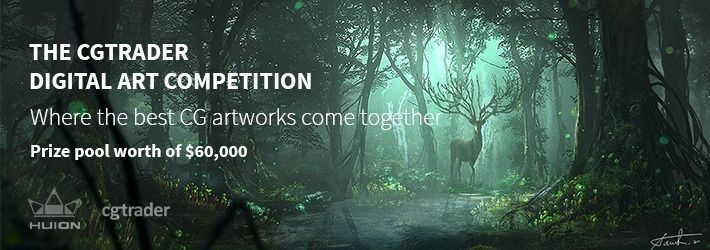-=+=- -=+=- -=+=- -=+=- -=+=- -=+=- -=+=- -=+=- -=+=- -=+=- -=+=- -=+=- -=+=- -=+=- -=+=- -=+=- -=+=- -=+=- -=+=- -=+=- -=+=- -=+=- -=+=- -=+=- -=+=- -=+=- -=+=- -=+=- -=+=- -=+=- (c) WidthPadding Industries 1987 0|349|0 -=+=- -=+=- -=+=- -=+=- -=+=- -=+=- -=+=- -=+=- -=+=- -=+=- -=+=- -=+=- -=+=- -=+=- -=+=- -=+=- -=+=- -=+=- -=+=- -=+=- -=+=- -=+=- -=+=- -=+=- -=+=- -=+=- -=+=- -=+=- -=+=- -=+=-
Socoder -> Concept/Design -> CGTrader's Digital Art Competition

Posted : Friday, 25 May 2018, 06:40CGTrader's Digital Art CompetitionHi guys,

CGTrader has just launched the Digital Art Competition with prizes worth \$60,000, and it’s open to all CG designers (both 2D and 3D)!

It’s easy – just submit your works of art to six very broad categories, for example: Illustration or Object Concept. There are no hard requirements, and artworks do not have to be created exclusively for the competition. We would be more than happy to see your submission in our contest!

In addition to the prizes, the competition offers massive exposure to artists in CGtrader's 1.4M+ user community, and the entry which gets the most votes will win the Public Award.

For more detailed requirements visit the competition page: www.cgtrader.com/digital-art-competition
Posted : Friday, 25 May 2018, 06:50
JayenkaiSince CGTrader registered a couple of days ago, I've been scouring the web for "Bad Stuff" and Spam warnings and the like. I was fairly sure the banhammer would be in good use.

But it's a compo. And I'm not going to block a compo, unless anyone else has any horror stories to tell about CGTrader.

Compo : Have-At

-=-=-